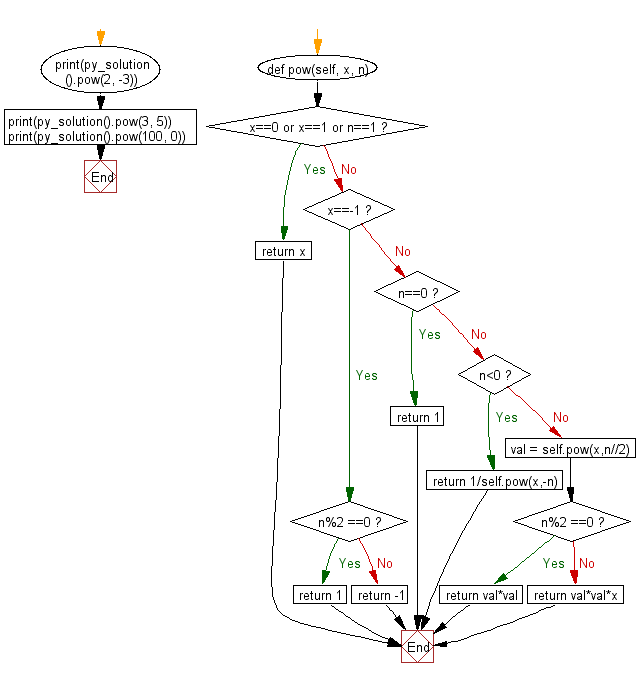﻿ Python: Implement pow(x, n) - w3resource# Python: Implement pow(x, n)

## Python Class: Exercise-7 with Solution

Write a Python class to implement pow(x, n).

Sample Solution:

Python Code:

``````class py_solution:
def pow(self, x, n):
if x==0 or x==1 or n==1:
return x

if x==-1:
if n%2 ==0:
return 1
else:
return -1
if n==0:
return 1
if n<0:
return 1/self.pow(x,-n)
val = self.pow(x,n//2)
if n%2 ==0:
return val*val
return val*val*x

print(py_solution().pow(2, -3));
print(py_solution().pow(3, 5));
print(py_solution().pow(100, 0));
```
```

Sample Output:

```0.125
243
1
```

Pictorial Presentation:Flowchart:## Visualize Python code execution:

The following tool visualize what the computer is doing step-by-step as it executes the said program:

Python Code Editor:

What is the difficulty level of this exercise?

Test your Programming skills with w3resource's quiz.

﻿

## Python: Tips of the Day

The Zip() Function:

```>>> students = ('John', 'Mary', 'Mike')
>>> ages = (15, 17, 16)
>>> scores = (90, 88, 82, 17, 14)
>>> for student, age, score in zip(students, ages, scores):
...     print(f'{student}, age: {age}, score: {score}')
...
John, age: 15, score: 90
Mary, age: 17, score: 88
Mike, age: 16, score: 82
>>> zipped = zip(students, ages, scores)
>>> a, b, c = zip(*zipped)
>>> print(b)
(15, 17, 16)
```